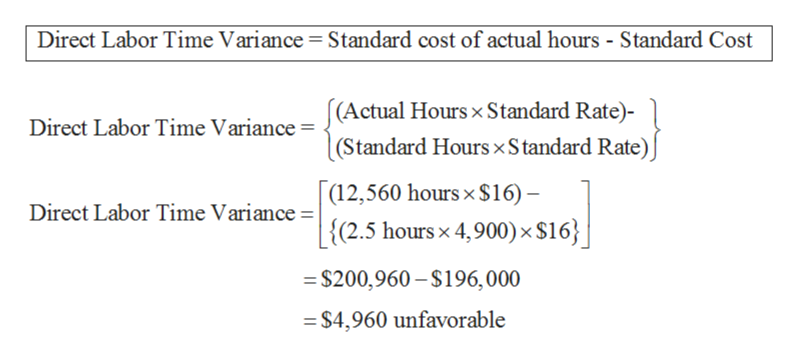# Peking Palace Company reported the following:Standard hours per unit2.5 hoursStandard rate per hour\$16.00Actual hours used12,560Actual rate per hour\$15.50Number of units produced4,900 What is the direct labor time variance?a.\$(4,805) favorableb.\$(4,960) favorablec.\$4,805 unfavorabled.\$4,960 unfavorable

Question
4 views
1. Peking Palace Company reported the following:

 Standard hours per unit 2.5 hours Standard rate per hour \$16.00 Actual hours used 12,560 Actual rate per hour \$15.50 Number of units produced 4,900

What is the direct labor time variance?

a.\$(4,805) favorable

b.\$(4,960) favorable

c.\$4,805 unfavorable

d.\$4,960 unfavorable

check_circle

Step 1

Direct labor Time Variance

Direct labor Efficiency variance is the measure of difference between the standard costs of actual number of direct labor hours utilized during a period and standard hours of direct labor for the level of output achieved.

Step 2

Following is the calculation of d...help_outlineImage TranscriptioncloseDirect Labor Time Variance = Standard cost of actual hours - Standard Cost Direct Labor Time Variance= (Actual Hours x Standard Rate)- (Standard Hours x Standard Rate) (12,560 hours x \$16)- {(2.5 hoursx 4,900) x \$16 | Direct Labor Time Variance = | \$200,960-\$196,000 =\$4,960 unfavorable fullscreen

### Want to see the full answer?

See Solution

#### Want to see this answer and more?

Solutions are written by subject experts who are available 24/7. Questions are typically answered within 1 hour.*

See Solution
*Response times may vary by subject and question.
Tagged in